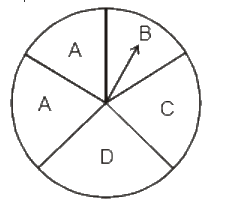# Ex.5.3 Q1 Data Handling Solutions - NCERT Maths Class 8

Go back to  'Ex.5.3'

## Question

List the outcomes you can see in these experiments.

(a) Spinning a wheel

(b) Tossing two coins togetherVideo Solution
Data Handling
Ex 5.3 | Question 1

## Text Solution

What is known?

Two different condition.

What is unknown?

Outcomes of the given conditions.

Reasoning:

All the possibilities of an event are known as its outcomes.

Steps:

(a) There are four letters $$A, B, C$$ and $$D$$ in a spinning wheel. So, there are $$4$$ outcomes.

(b) When two coins are tossed together there are four possible outcomes

$$HH, HT, TH, TT.$$

(Here $$HT$$ means head on first coin and tail on second coin and so on.)

Learn from the best math teachers and top your exams

• Live one on one classroom and doubt clearing
• Practice worksheets in and after class for conceptual clarity
• Personalized curriculum to keep up with school